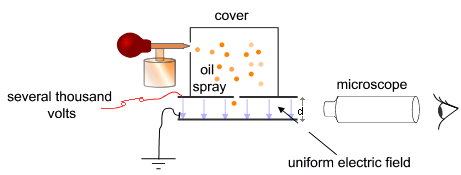Millikan’s Oil Drop Experiment

Learning Objective

• Describe the major findings of Millikan’s oil drop experiment

Key Points

• Millikan’s oil drop experiment measured the charge of an electron. Before this experiment, existence of subatomic particles was not universally accepted.
• Millikan’s apparatus contained an electric field created between a parallel pair of metal plates, which were held apart by insulating material. Electrically charged oil droplets entered the electric field and were balanced between two plates by altering the field.
• When the charged drops fell at a constant rate, the gravitational and electric forces on it were equal. Therefore, the charge on the oil drop was calculated using formula Q = $\frac {m\cdot g}{E}$ Millikan found that the charge of a single electron was 1.6 x 10-19 C.

Terms

• oil drop experimentExperiment performed by Robert Millikan and Harvey Fletcher in 1909 to measure the charge of the electron.
• electronThe subatomic particle having a negative charge and orbiting the nucleus; the flow of electrons in a conductor constitutes electricity.

The Oil Drop Experiment

In 1909, Robert Millikan and Harvey Fletcher conducted the oil drop experiment to determine the charge of an electron. They suspended tiny charged droplets of oil between two metal electrodes by balancing downward gravitational force with upward drag and electric forces. The density of the oil was known, so Millikan and Fletcher could determine the droplets’ masses from their observed radii (since from the radii they could calculate the volume and thus, the mass). Using the known electric field and the values of gravity and mass, Millikan and Fletcher determined the charge on oil droplets in mechanical equilibrium. By repeating the experiment, they confirmed that the charges were all multiples of some fundamental value. They calculated this value to be 1.5924 × 10−19Coulombs (C), which is within 1% of the currently accepted value of 1.602176487 × 10−19 C. They proposed that this was the charge of a single electron.

How did the process work?

The figure below shows a simplified scheme of Millikan’s oil drop experiment. The apparatus incorporated a pair of metal plates and a specific type of oil. Millikan and Fletcher discovered it was best to use an oil with an extremely low vapor pressure, such as one designed for use in a vacuum apparatus. Ordinary oil would evaporate under the heat of the light source, causing the mass of the oil drop to change over the course of the experiment.

By applying a potential difference across a parallel pair of horizontal metal plates, a uniform electric field was created in the space between them. A ring of insulating material was used to hold the plates apart. Four holes were cut into the ring—three for illumination by a bright light and another to allow viewing through a microscope. A fine mist of oil droplets was sprayed into a chamber above the plates. The oil drops became electrically charged through friction with the nozzle as they were sprayed. Alternatively, charge could be induced by including an ionizing radiation source (such as an X-ray tube).Simplified scheme of Millikan’s oil-drop experimentThis apparatus has a parallel pair of horizontal metal plates. A uniform electric field is created between them. The ring has three holes for illumination and one for viewing through a microscope. A specific type of oil is sprayed into the chamber, where drops become electrically charged. The droplets enter the space between the plates and can be controlled by changing the voltage across the plates.

The droplets entered the space between the plates and, because they were charged, they could be controlled by changing the voltage across the plates. Initially, the oil drops were allowed to fall between the plates with the electric field turned off. They quickly reached terminal velocity due to friction with the air in the chamber. The field was turned on and, if it was large enough, some of the drops (the charged ones) would start to rise. This is because the upwards electric force, FE, is greater for them than the downwards gravitational force, g. (A charged rubber rod can pick up bits of paper in the same way.) A likely looking drop was selected and kept in the middle of the field of view by alternately switching off the voltage until all the other drops fell. The experiment was continued with this single drop.

Millikan’s experiment was meant to have the drops fall at a constant rate. At this constant rate, the force of gravity on the drop and the force of the electric field on the drop are equal:

Fup = Fdown

$\cdot$Fup = Q$\cdot$E Fdown = m$\cdot$

Q is the charge of an electron, E is the electric field, m is mass of the droplet, and g is gravity.

Q$\cdot$E = m$\cdot$g

Q = $\frac{m\cdot g}{E}$

One can see how Millikan calculated the charge of an electron. Millikan found that all drops had charges which were multiples of 1.6 x 10-19 C.

At the time of Millikan and Fletcher’s oil drop experiments, the existence of subatomic particles was not universally accepted. Experimenting with cathode rays in 1897, J. J. Thomson discovered negatively charged “corpuscles” with a mass about 1,840 times smaller than that of a hydrogen atom. George FitzGerald and Walter Kaufmann found similar results. In 1923, Millikan won the Nobel Prize in physics in part because of this experiment.

Aside from discerning an electron’s charge, the beauty of the oil drop experiment lies in its simple and elegant demonstration that charge is actually quantized. The experiment has since been repeated by generations of physics students—although it is rather expensive and difficult to do properly.